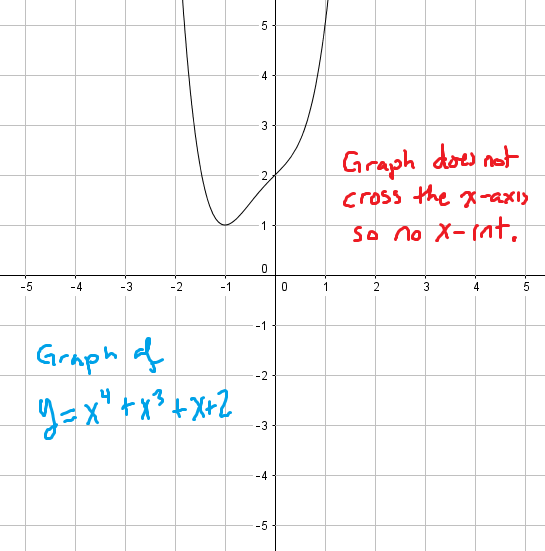﻿ Finding the x-intercepts of a function - Math Bootcamps

# Finding and understanding x-intercepts

Given the graph of any function, an x-intercept is simply the point, or points where the graph crosses the x-axis. There might be just one such point, no such point, or many, meaning a function can have several x-intercepts. As you will see below, we can use a graph or a simple algebra rule to find the x-intercept or x-intercepts of any function. You can also scroll down to a video example below.

## Finding the x-intercept or x-intercepts using a graph

As mentioned above, functions may have one, zero, or even many x-intercepts. These can be found by looking at where the graph of a function crosses the x-axis, which is the horizontal axis in the xy-coordinate plane. You can see this on the graph below. This function has a single x-intercept.In the graph below, the function has two x-intercepts. Notice that the form of the point is always $$(c, 0)$$ for some number $$c$$.Finally, the following graph shows a function with no x-intercepts. You can see this because it does not cross the x-axis at any point.You can see a more advanced discussion of these ideas here: The zeros of a polynomial.

## Finding the x-intercept or intercepts using algebra

The general rule for finding the x-intercept or intercepts of any function is to let $$y = 0$$ and solve for $$x$$. This may be somewhat easy or really difficult, depending on the function. Let’s look at some examples to see why this may be the case.

### Example

Find the x-intercept of the function: $$y = 3x – 9$$

### Solution

Let $$y = 0$$ and solve for $$x$$.

\begin{align}0 &= 3x – 9\\ -3x &= -9\\x &= 3\end{align}

Answer: Therefore the x-intercept is 3. You could also write it as a point: $$(3,0)$$

A more complicated example would be one where the equation representing the function itself is more complex. For these situations, you need to know a little more algebra in order to find any intercepts.

### Example

Find the x-intercepts for the function: $$y = x^2 + 2x – 8$$

### Solution

As before, let $$y = 0$$ and solve for $$x$$. This time, you have a quadratic equation to solve.

\begin{align} 0 &= x^2 + 2x – 8\\ 0 &= (x + 4)(x – 2)\\ x &= -4, 2\end{align}

Answer: This function has two x-intercepts: –4 and 2. These are located at $$(–4, 0)$$ and $$(2, 0)$$.

For equations more complex than this, a graphing calculator is often useful for at least estimating the location of any intercepts.

## Video examples

In the following video, you can see how to find the x-intercepts of three different functions. This also includes an example where there are no x-intercepts.

## Continue your study of graphs

You can continue your study of graphing with the following articles.## Subscribe to our Newsletter!

We are always posting new free lessons and adding more study guides, calculator guides, and problem packs.

Sign up to get occasional emails (once every couple or three weeks) letting you know what's new!